# Relative and Absolute Cell References in Microsoft Excel

A cell reference refers to a single cell or range of cells on a Excel worksheet. These cells can be referred to by Excel Formulas when calcuations are made. In this tutorial you will learn the differences between relative and absolute cell references. Each behaves differently when copied and filled to other cells. Relative references change when a formula is copied to another cell whereas Absolute references, remain constant, no matter where they are copied.

For more on hands-on Excel training classes in Los Angeles call us on 888.815.0604.

#### Understanding Relative and Absolute Cell References

Excel worksheets are composed of rows (horizontal, referenced by numbers) and columns (vertical, referenced by letters). When these two elements intersect, they create a cell, and each cell is given a name. In the example below, you can see that the cell that is selected is named “C2.” This is because it lies at the intersection of column C and row 2: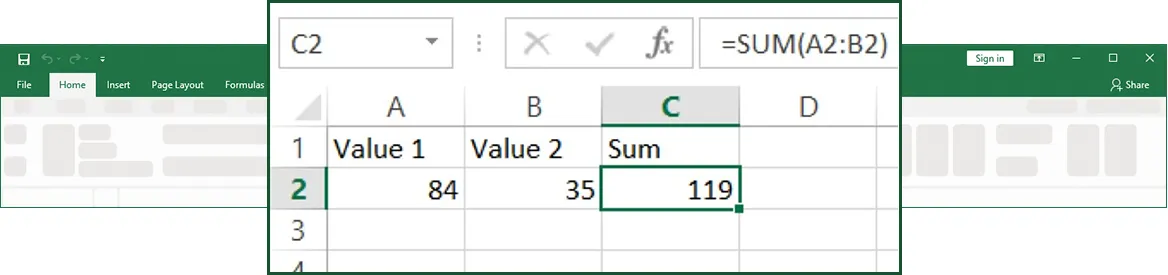This particular cell contains a formula that adds the values found in cells A2 and B2 together, which you can see in the formula bar when cell C2 is selected. Select cell C2. Click and drag the small square in the bottom right-hand corner of this cell down one cell to C3: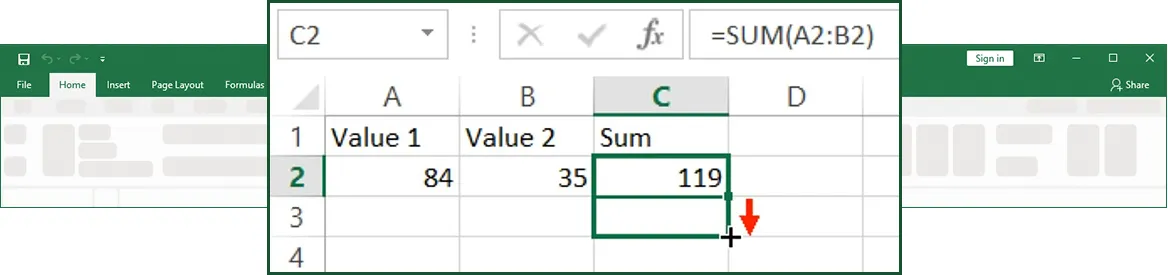Release your mouse button. You will see that cell C3 now contains 0: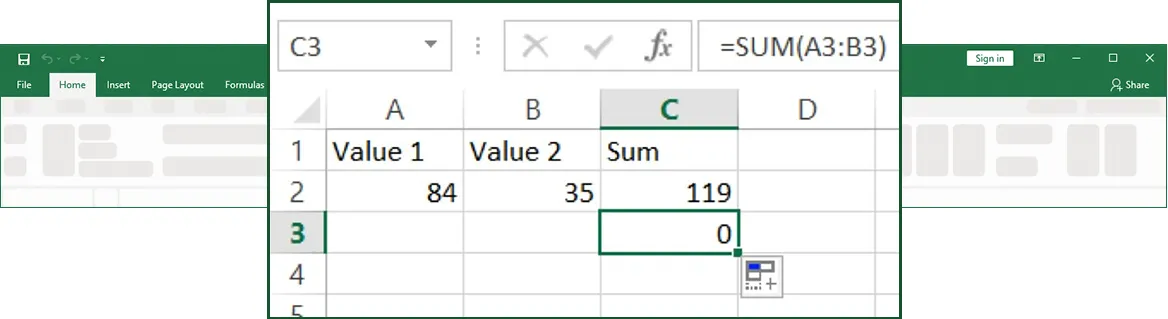This has happened because the cell references, which are visible in the formula bar when C3 is selected, have been changed. When the AutoFill operation was performed, the cell references were modified relative to the location of the formula. Since A3 and B3 contain no values, the result is 0.

The same thing will happen if you copy and paste the contents of cell C2 somewhere else. Try pasting cell C2 to F4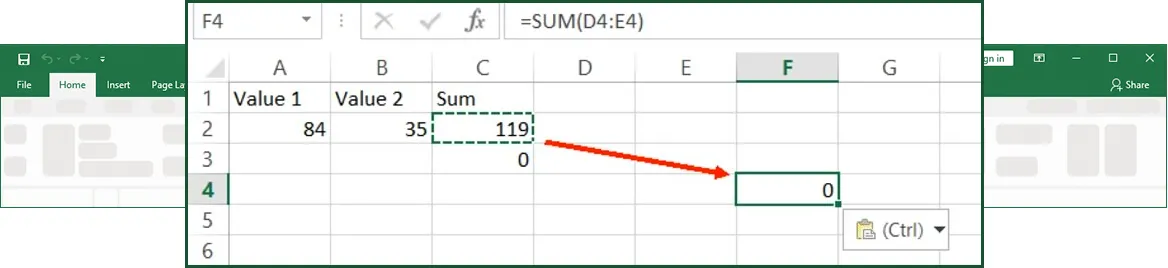F4 will now display a value of 0 because the values relative to the formula location (D4 and E4) are empty. This happens because the original formula uses relative cell references. Most of the time, these relative references aren’t an issue. However, if data is to be moved around or copied using AutoFill, relative cell references can create incorrect and confusing results.

To avoid this, you can use absolute cell references. These references use dollar signs (\$) to make sure a formula always references the same location, no matter where it is moved. You can assign absolute cell references in three ways:

• \$Column\$Row: Both the row and column designation won’t change (\$A\$1).
• \$ColumnRow: The column designation won’t change, but the row can (\$A1).
• Column\$Row: The row designation won’t change, but the column can (A\$1).

Select cell C2. Replace the formula with the following formula that uses absolute cell references: =\$A\$2+\$B\$2: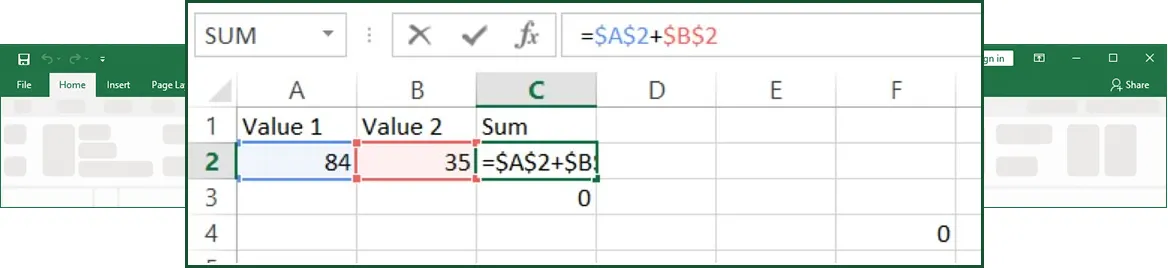Press Enter to apply the new changes. Copy and paste this cell to E3. You will see that the formula and result will stay the same: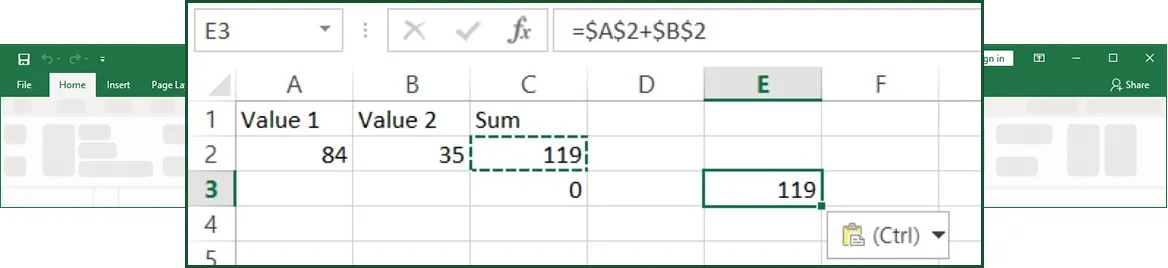Save the changes that you have made to the current workbook and then close Microsoft Excel.

#### Onsite Excel training

Through our network of local trainers we deliver onsite Microsoft Excel throughout the country. View our Excel testimonials or obtain a quote for a customized Excel class.